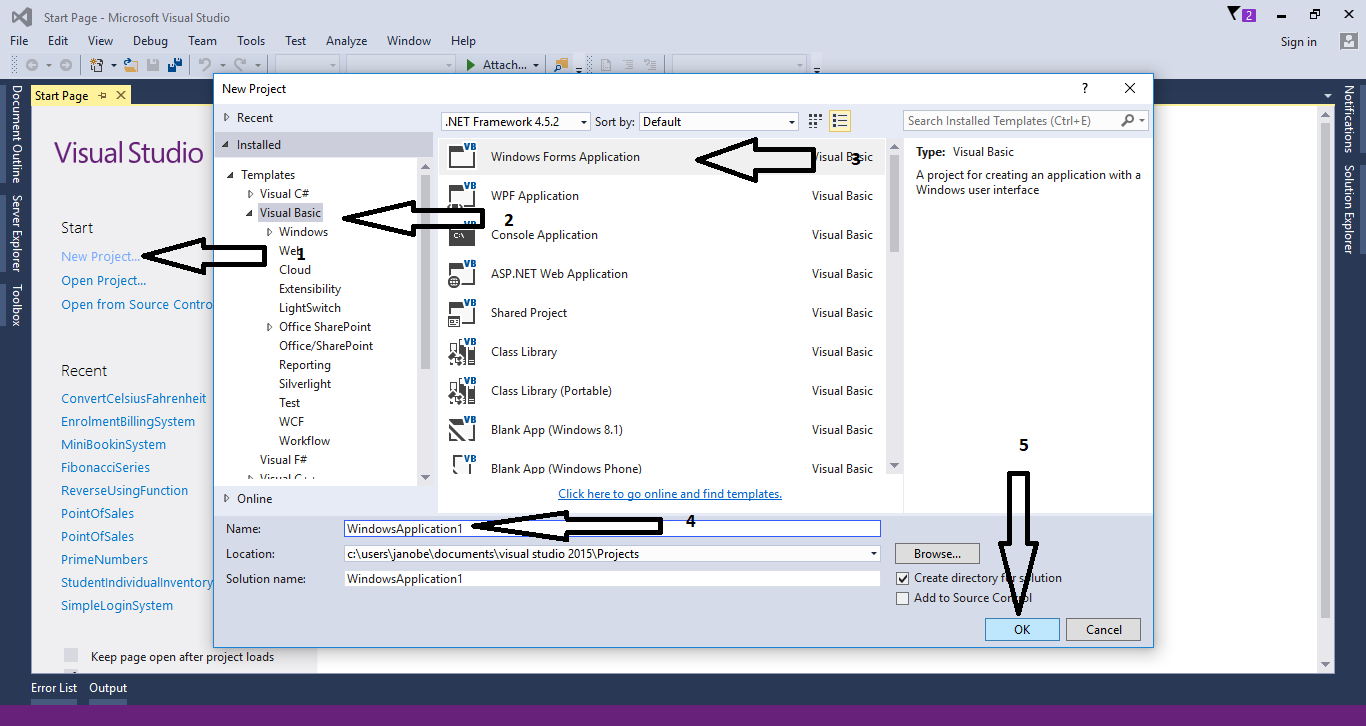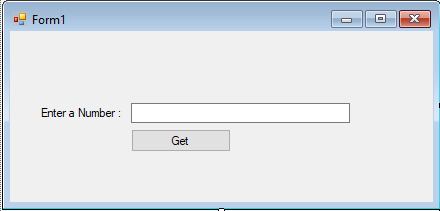# How to Get Factorial Numbers in VB.Net

Body
This time, let’s learn how to get factorial numbers in VB.Net. If we say factorial number, it means a number which is multiply itself to its decreasing numbers and it is denoted by the symbol (!). Like for example the factorial number or value of 5. This is how it looks like, 5! = 5 x 4 x 3 x 2 x 1 = 120. Now, let’s try doing this with codes in VB.Net. Just simply follow the instructions below.

## Step 1

Open Microsoft Visual Studio 2015 and create a new windows form application.## Step 2

Add the Label, Button and a TextBox inside the Form.## Step 3

Double click the button and do the following code inside the sub procedure for getting factorial numbers.
`        Dim n, f, i As Integer        f = 1        n = Val(TextBox1.Text)        For i = 1 To n            f = f * i        Next        MsgBox(“Factorial is :” & f, vbInformation, “Factorial”)`